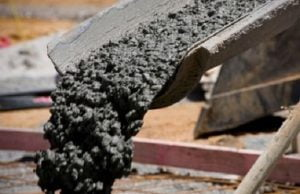Tuesday, October 3, 2023

# Water Required for M20 Concrete, 50 Kg of Cement | M15, M25 Concrete

In this article, we will discuss the water required for M20 concrete.

### 1. Introduction

Concrete is the composite material that is composed of a mixture of fine and coarse aggregates bonded together with biding material which hardens over time. The binding material mostly includes fluid cement paste, lime putty, lime, etc.

The Strength & workability of concrete varies with the water/ cement ratio which comes under 0.4 to 0.6 according to the IS456 codebook for the nominal mix.

The water-cement ratio may be defined as the ratio between the weight of water to the weight of cement, it is usually denoted by the w/c ratio.In general terms, it is explain how much water it needs for a particular mix. As the water-cement ratio hugely influences the strength of concrete it can also influence the workability and consistency of concrete.

There are various grades of concrete for example M5, M7.5, M10, M15, M20, M25 divided according to their compressive strength. Here, M stands for mix and number denotes the compressive strength.

Water cement ratio will directly influence the strength, the proper portion will increase the strength of concrete but, in case of improper proportion, the strength will decrease.

### 2. M15 Concrete

The M15 concrete represents a mixture of cement, sand, and aggregate which are prepared by making a cement concrete cube of size 15cm x 15cm x 15cm is made with the characteristic of compressive strength (fck) of 15 MPa or 15 N/mm2 while testing it after being cured for 28 days. The usually considered nominal mix ratio for M15 concrete is 1:2:4 (1 part cement to 2 parts sand and to 4 parts aggregate).

### 3. M20 Concrete

The M20 concrete gives a mixture of cement, sand, and aggregate which are made in such a way that a cement concrete cube of size 15 cm x 15 cm x 15 cm is formed with characteristic of compressive strength (fck) of 20 MPa or 20 N/mm2 while examining it after being cured for 28 days. The nominal mix ratio for M20 concrete is taken as 1:1.5:3 (1 part cement to 1.5 parts sand and to 3 parts aggregate).

### 4. M25 Concrete

The M25 concrete represents a mixture of cement, sand, and aggregate which are prepared in such a manner that a cement concrete cube of size 15cm x 15cm x 15cm is formed with characteristic of compressive strength (fck) of 25 MPa or 25 N/mm2 while examining it after being cured for 28 days. The normally considered nominal mix ratio for M25 concrete is 1:1:2 (1 part cement to 1 parts sand and to 2 parts aggregate).

Every Kg of cement requires around a minimum of 0.40 liters, a maximum of 0.60 liters. It majorly depends upon the type of concrete grade, weather condition, and strength of concrete needed.

### 5. Water Required for M20 Concrete

The quantity of water required for M20 concrete per 50kg bag of cement for a normal mix of concrete based on the water-cement ratio which comes under 0.4 to 0.6, means every 1kg of cement will require a minimum of 0.4 liters of water and a maximum of about 0.6 liters, therefore, for a 50kg bag of cement water needed is about 20 to 30 liters on various exposure condition.

The minimum water quantity per 50kg cement = 50×0.4 = 20 litres,

&

The maximum water quantity per 50kg cement = 50×0.6 = 30 litres.

### 6. Calculation of Water Required For M20 Concrete

Here are the steps followed to calculate the water required for M20 concrete are as follows:

1. Take a wet quantity of a nominal mix of M20 concrete is about 1m3, so dry volume of concrete = 1× 1.54 = 1.54 m3

calculate part of cement in the mix, total proportion such as 1 +1.5+3 = 5.5, then the amount of cement = 1/5.5 of dry volume

2. Calculate needed cement quantity = 1/5.5 × 1.54 × 1440 kg/m3 = 403 kg

3. Calculate the needed amount of water, as 0.55 is the w/c ratio for m20 concrete, so the amount of water needed for 1m3 of a nominal mix of m20 concrete is about 240 liters, calculation such as 403 × 0.55 = 220 liters.

As per the IS456 codebook, in mild exposure conditions w/c ratio for a normal mix of M20 concrete is about 0.55, so for a 50 kg bag of cement water required is about 28 liters. The calculation of water quantity = (220 ÷ 403)× 50 = 28 litres.

The maximum quantity of water needed per 50kg bag of cement for a nominal mix of M20 grade of concrete is about 30 liters on mild exposure environmental conditions. Maths calculation such as maximum w/c ratio is 0.60, water quantity for 403kg cement = 403× 0.6 = 240 litres, so water required per 50kg cement = (240÷403) × 50 = 30 litres.

The minimum quantity of water needed per 50kg bag of cement for a nominal mix of M20 grade of concrete is about 20 liters on extreme exposure environmental conditions. The calculation is done on such a away that  minimum w/c ratio is 0.40, water quantity for 403 kg cement = 403× 0.4 = 160 litres, so water required per 50 kg cement = (160÷403) × 50 = 20 litres.He is a founder and lead author of Dream Civil International and his civil engineering research articles has been taken as source by world's top news and educational sites like USA Today, Time, The richest, Wikipedia, etc.
Latest Articles

Related Articles